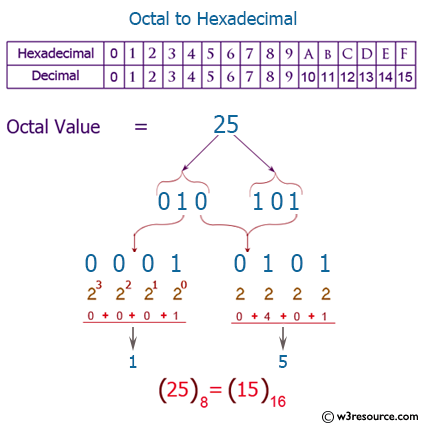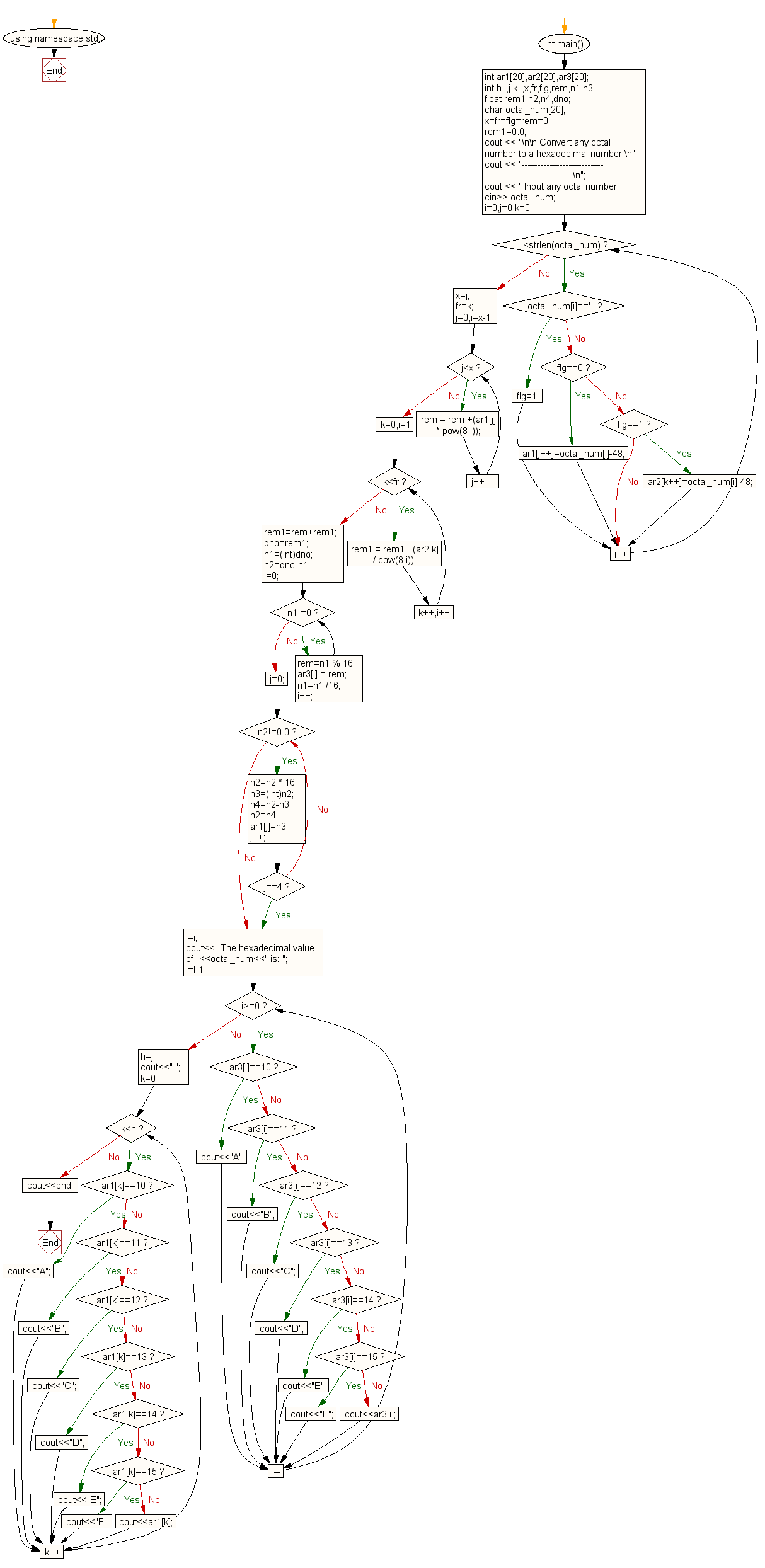﻿ C++ Exercises: Convert a octal number to a hexadecimal number - w3resource# C++ Exercises: Convert a octal number to a hexadecimal number

## C++ For Loop: Exercise-78 with Solution

Write a program in C++ to convert a octal number to a hexadecimal number.

Pictorial Presentation:Sample Solution:-

C++ Code :

``````#include<iostream>
#include<string.h>
#include<math.h>
using namespace std;

int main()
{
int ar1,ar2,ar3;
int h,i,j,k,l,x,fr,flg,rem,n1,n3;
float rem1,n2,n4,dno;
char octal_num;
x=fr=flg=rem=0;
rem1=0.0;
cout << "\n\n Convert any octal number to a hexadecimal number:\n";
cout << "------------------------------------------------------\n";
cout << " Input any octal number: ";
cin>> octal_num;

for(i=0,j=0,k=0;i<strlen(octal_num);i++)
{
if(octal_num[i]=='.')
{
flg=1;
}
else if(flg==0)
ar1[j++]=octal_num[i]-48;
else if(flg==1)
ar2[k++]=octal_num[i]-48;
}
x=j;
fr=k;
for(j=0,i=x-1;j<x;j++,i--)
{
rem = rem +(ar1[j] * pow(8,i));
}
for(k=0,i=1;k<fr;k++,i++)
{
rem1 = rem1 +(ar2[k] / pow(8,i));
}
rem1=rem+rem1;
dno=rem1;
n1=(int)dno;
n2=dno-n1;

i=0;
while(n1!=0)
{
rem=n1 % 16;
ar3[i] = rem;
n1=n1 /16;
i++;
}
j=0;
while(n2!=0.0)
{
n2=n2 * 16;
n3=(int)n2;
n4=n2-n3;
n2=n4;
ar1[j]=n3;
j++;
if(j==4)
{
break;
}
}
l=i;
cout<<" The hexadecimal value of "<<octal_num<<" is: ";
for(i=l-1;i>=0;i--)
{
if(ar3[i]==10)
cout<<"A";
else if(ar3[i]==11)
cout<<"B";
else if(ar3[i]==12)
cout<<"C";
else if(ar3[i]==13)
cout<<"D";
else if(ar3[i]==14)
cout<<"E";
else if(ar3[i]==15)
cout<<"F";
else
cout<<ar3[i];
}
h=j;
cout<<".";
for(k=0;k<h;k++)
{
if(ar1[k]==10)
cout<<"A";
else if(ar1[k]==11)
cout<<"B";
else if(ar1[k]==12)
cout<<"C";
else if(ar1[k]==13)
cout<<"D";
else if(ar1[k]==14)
cout<<"E";
else if(ar1[k]==15)
cout<<"F";
else
cout<<ar1[k];
}
cout<<endl;
}
``````

Sample Output:

``` Convert any octal number to a hexadecimal number:
------------------------------------------------------
Input any octal number: 77
The hexadecimal value of 77 is: 3F.
```

Flowchart:C++ Code Editor:

Contribute your code and comments through Disqus.

What is the difficulty level of this exercise?

﻿

## C++ Programming: Tips of the Day

What is a smart pointer and when should I use one?

This answer is rather old, and so describes what was 'good' at the time, which was smart pointers provided by the Boost library. Since C++11, the standard library has provided sufficient smart pointers types, and so you should favour the use of std::unique_ptr, std::shared_ptr and std::weak_ptr.

There was also std::auto_ptr. It was very much like a scoped pointer, except that it also had the "special" dangerous ability to be copied - which also unexpectedly transfers ownership.

It was deprecated in C++11 and removed in C++17, so you shouldn't use it.

```std::auto_ptr<MyObject> p1 (new MyObject());
std::auto_ptr<MyObject> p2 = p1; // Copy and transfer ownership.
// p1 gets set to empty!
p2->DoSomething(); // Works.
p1->DoSomething(); // Oh oh. Hopefully raises some NULL pointer exception.
```

Ref : https://bit.ly/3mc9GHE